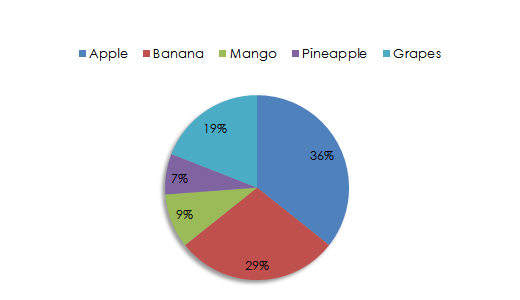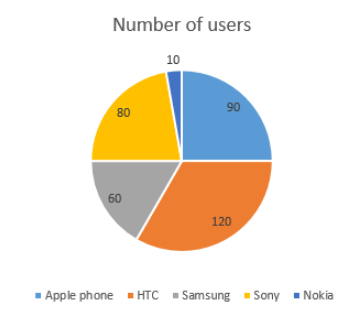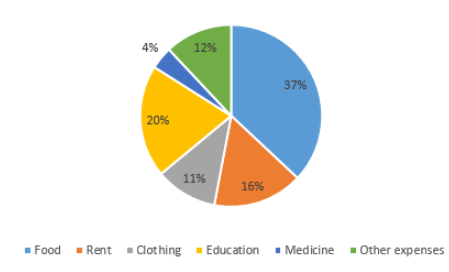# Circle Graph Formula

In graph theory, a circle graph is the intersection graph of a set of chords of a circle. It is an undirected graph whose vertices can be associated with chords of a circle such that two vertices are adjacent if and only if the corresponding chords cross each other. The circle graph is also called a pie graph and pie chart.

Some important formulas of circle graph are given below:

1. Percentages = (Amount in the Category ⁄ Total) × 100

2. Angle = (Amount in the Category ⁄ Total) × 360°

### Solved Examples

Example 1: A fruit basket is filled with 36% Apples, 29% Bananas, 9% Mangoes, 7% Pineapples and 19% Grapes. Prepare a circle graph chart as per the data provided.

Solution: Given that,
Apples – 36%
Bananas – 29%
Mangoes – 9%
Pineapples – 7%
Grapes – 19%
With the above data, the circle graph will be drawn as:Example 2: The following data gives information about the number of different mobile phones used by the employees of one department in a company. Draw the circle chart for the following data using angle proportions.

 Mobile phone Number of users Apple phone 9 HTC 12 Samsung 6 Sony 8 Nokia 1

Solution: Angle = (Amount in the Category ⁄ Total) × 360°

 Mobile phone Number of users Angle Apple phone 9 (9/36) × 360° = 90° HTC 12 (12/36) × 360° = 120° Samsung 6 (6/36) × 360° = 60° Sony 8 (8/36) × 360° = 80° Nokia 1 (1/36) × 360° = 10°Example 3: The below table shows the percentage of expenses of a person in a month. Draw a circle chart for the data given.

 Category Percentage of expense Food 37% Rent 16% Clothing 11% Education 20% Medicine 4% Other expenses 12%

Solution:

Given that,
Food = 37%
Rent = 16%
Clothing = 11%
Education = 20%
Medicine = 4%
Other expenses = 12%